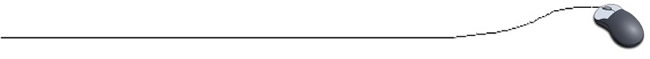Controlling Decimal Output

It is often necessary to print values with a designated number of decimal places.  For example, when printing amounts of money, it is customary to have two decimal places printed to the right of the decimal point.  The money amounts of  \$15.1 and  \$53.9 are more commonly seen as  \$15.10 and  \$53.90.

There are many ways to accomplish printing decimal money amounts (and other decimal values) by using a printing format system. Our first option, to insure that a designated number of decimal places will be printed, is to utilize the DecimalFormat Class.  When using the DecimalFormat Class, it ill be necessary to include:

import java.text.*;

You will need to create an object of the class DecimalFormat.
DecimalFormat  variable_name =  new DecimalFormat("000.000");

The String pattern will designate how your display will look.  The number of zeros in front of the decimal point will be the minimum number of locations printed to the left of the decimal.  The number of zeros behind the decimal point will be the maximum number of locations printed to the right of the decimal. A "strange" version of rounding will occur.  With the pattern shown above, the number 45.66874 would print as 045.669 (normal rounding).

 Sample program: import java.text.*; public class DecimalTest {     public static void main(String[ ] args)  {         double money = .159;         double mula = 36.5;         double mula2 = 443336.333                    DecimalFormat decFor = new DecimalFormat("\$#,###.00");         System.out.println(decFor.format(money)+"\n"+                decFor.format(mula) +"\n"+ decFor.format(mula2));      } } Screen output: \$.16 \$36.50 \$443,336.33
 So, why did we say the rounding was "strange"? These examples look normal. But, unfortunately, not all situations will look normal. For example, when rounding to nearest integer: 1.5 rounds to 2, while 2.5 rounds to 2. This rounding is certainly not what we think of as "normal" rounding. The DecimalFormat uses half-even rounding for formatting. The number is rounded up, or rounded down, if the number is nearer to the next neighbor (normal rounding). When the number is "exactly" between two neighbors (such as 2.5 is exactly between 2 and 3), the extra decimal places are truncated (dropped off) when the preceding digit is even (2.5 rounds to 2). If the preceding digit is odd, it rounds to the nearest even number (normal rounding, 3.5 rounds to 4). This type of rounding is known as "banker's rounding." The supposed advantage is that this method is unbiased when used in the extreme allowing the average of error to approach zero. We will see that Math.round will be our choice for "normal" rounding. Remember that the "printf" formatting pattern also rounds.

More possible String patterns:
In all of the following patterns, the computer will round the decimal value to the number of indicated decimal places. If 0's appear in the pattern, trailing 0's will be filled in when needed. If #'s appear in the pattern, no trailing 0's will be filled in the pattern.

• forcing leading and trailing 0's to appear if no values are present: 000.000

• place "comma" separations in larger numbers and 2 decimal places rounded: #,###.##
(no forced trailing 0's)

• place a dollar sign immediately before leftmost digit: \$#,###.## (no forced trailing 0's)

• combinations of patterns will also work: \$#,###.00 (trailing 0's will appear if needed, no leading 0)

• a good choice for money: \$#,##0.00 (both trailing and leading 0 when needed)
double money = .159; would be displayed as \$0.16.

 Things to Remember about the DecimalFormat class: 1. it needs import.java.text.*; 2. the pattern (such as "00.00") establishes how the output will look. 3. it rounds by "banker's rounding". 4. it is necessary to specify EACH value you wish printed in this formatted pattern.